You are here: Start » AVL.NET » Path Features

# Path Features

IconNameDescription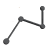PathArrayPointsConverts an array of paths to an array of points.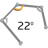PathAverageTurnAngleComputes the average absolute turn angle of a path per unit of length.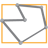PathBoundingBoxComputes the smallest box containing a path.PathBoundingBox_OrNilComputes the smallest box containing a path; returns NIL if the path is empty.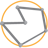PathBoundingCircleComputes the smallest circle enclosing a path.PathBoundingCircle_OrNilComputes the smallest circle enclosing a path; returns NIL if the path is empty.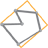PathBoundingParallelogramComputes the smallest parallelogram containing a path.PathBoundingRectangleComputes the smallest rectangle containing a path.PathBoundingRectangle_FixedAngleComputes the smallest rectangle with the given orientation angle containing a path.PathBoundingRectangle_FixedAngle_OrNilComputes the smallest rectangle with the given orientation angle containing a path; returns NIL when the path is empty.PathBoundingRectangle_OrNilComputes the smallest rectangle containing a path; returns NIL when the path is empty.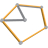PathConvexHullComputes the smallest convex shape that contains the given path.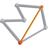PathDiameterFinds the longest segment connecting two characteristic points of a path.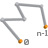PathEndpointsReturns the two endpoints of a path.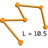PathLengthComputes the total length of the input path.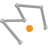PathMassCenterComputes the average of the path points (all, not only characteristic ones).PathMassCenter_OrNilComputes the average of the path points (all, not only characteristic ones); returns NIL if the path is empty.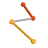PathSegmentsConverts a path to an array of line segments.PathSelfIntersectionsChecks if a path has any self-intersections and computes all of them.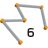PathSizeReturns the number of characteristic points on the input path.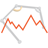PathTurnAngleLocalMaximaDetection of feature points related to object corners.PathTurnAngleMaximumFinds the maximum of the profile of turn angles of a path.PathTurnAngleMaximum_OrNilFinds the maximum of the profile of turn angles of a path; returns NIL if the path has no turn angles.PathTurnAngleProfileComputes the profile of turn angles at characteristic points of a path.